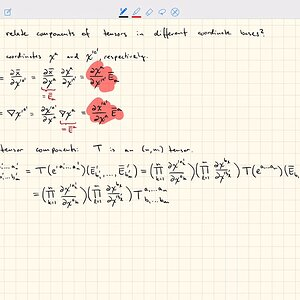# Coordinate transformations Definition and 8 Discussions

In geometry, a coordinate system is a system that uses one or more numbers, or coordinates, to uniquely determine the position of the points or other geometric elements on a manifold such as Euclidean space. The order of the coordinates is significant, and they are sometimes identified by their position in an ordered tuple and sometimes by a letter, as in "the x-coordinate". The coordinates are taken to be real numbers in elementary mathematics, but may be complex numbers or elements of a more abstract system such as a commutative ring. The use of a coordinate system allows problems in geometry to be translated into problems about numbers and vice versa; this is the basis of analytic geometry.

View More On Wikipedia.org
1. ### Coordinate Transformation (multivariable calculus)

My Progress: I tried to perform the coordinate transformation by considering a general function ##f(\mathbf{k},\omega,\mathbf{R},T)## and see how its derivatives with respect all variable ##(\mathbf{k},\omega,\mathbf{R},T)## change:  \frac{\partial}{\partial\omega} f =...
2. ### I Coordinate Transformation in a de Sitter Space?

Could one derive a set of coordinate transformations that transforms events between different reference frames in the de Sitter metric using the invariant line element, similar to how the Lorentz Transformations leave the line element of the Minkowski metric invariant? Would these coordinate...
3.4. ### General relativity- Coordinate/metric transformations

Homework Statement Consider the metric ds2=(u2-v2)(du2 -dv2). I have to find a coordinate system (t,x), such that ds2=dt2-dx2. The same for the metric: ds2=dv2-v2du2. Homework Equations General coordinate transformation, ds2=gabdxadxb The Attempt at a Solution I started with a general...
5. Q

### I Coordinate transformation - Rotation

How author derives these old basis unit vectors in terms of new basis vectors ? Please don't explain in two words. \hat{e}_x = cos(\varphi)\hat{e}'_x - sin(\varphi)\hat{e}'_y \hat{e}_y = sin(\varphi)\hat{e}'_x + cos(\varphi)\hat{e}'_y
6. ### A question concerning Jacobians

Apologies for perhaps a very trivial question, but I'm slightly doubting my understanding of Jacobians after explaining the concept of coordinate transformations to a colleague. Basically, as I understand it, the Jacobian (intuitively) describes how surface (or volume) elements change under a...
7. ### Coordinate transformation - NED and ECEF frames

Hi, I have a reference device that outputs euler angles, which are angles that relate the sensor body frame to the north east down frame. These angles are called pitch roll and yaw. The sensor is an accelerometer. I know how to get the rotation matrix that will put accelerations from the...
8. ### Question about Coordinate Change

Suppose that I have a two-dimensional coordinate system (x,y) and I change to a new coordinate system (u,v). What I know is that there is some function \theta(u,v) such that: \dfrac{\partial x}{\partial u} = cos(\theta) \dfrac{\partial x}{\partial v} = -sin(\theta) \dfrac{\partial y}{\partial...